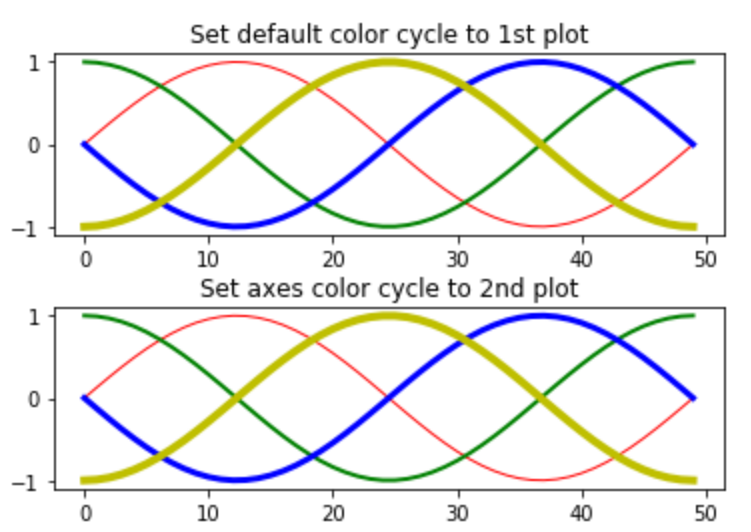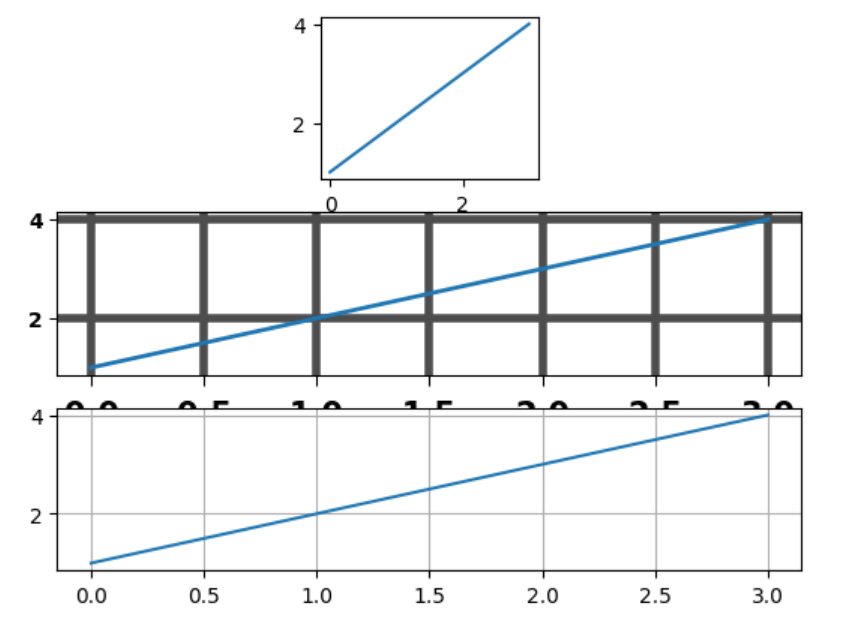# Matplotlib.pyplot.rc() in Python

Matplotlib is an amazing visualization library in Python for 2D plots of arrays. Matplotlib is a multi-platform data visualization library built on NumPy arrays and designed to work with the broader SciPy stack.

## matplotlib.pyplot.rc()

matplotlib.pyplot.rc() function is used to the rc params. Grouping in rc is done through the ‘group'(eg, for lines). For lines in axes the group is linewidth. The group for axes is facecolor and so on. A list or tuple can also act as a group name(eg, xtick, ytick). Kwargs act as a name value pair which broadly is a dictionary, eg:

Syntax:

rc(‘lines’, linewidth=3, color=’g’)

It sets the current rc params and is same as

rcParams[‘lines.linewidth’] = 3
rcParams[‘lines.color’] = ‘g’

To save typing for interactive users the following aliases are available:

Alias Property
‘lw’ ‘linewidth’
‘ls’ ‘linestyle’
‘c’ ‘color’
‘fc’ ‘facecolor’
‘ec’ ‘edgecolor’
‘mew’ ‘markeredgewidth’
‘aa’ ‘antialiased’

Therefore once could abbreviate the above rc commands as follows

rc(‘lines’, lw=3, c=’g’)

Note: One can use the pythons kwargs dictionary to store dictionaries of its default parameters. For example,

font = {‘family’ : ‘monospace’,
‘weight’ : ‘italic’,
‘size’ : ‘medium’}
# pass in the font dict as kwargs
rc(‘font’, **font)

This helps in easily switching among different configurations. you can also use matplotlib.style.use(‘default’) or rcdefaults() to restore back the rc params after change.

Example 1:

 `from` `cycler ``import` `cycler ` `import` `numpy as np ` `import` `matplotlib.pyplot as plt ` ` `  ` `  `# setting up a custom cycler ` `sample_cycler ``=` `(cycler(color ``=``[``'r'``, ``'g'``,  ` `                                ``'b'``, ``'y'``]) ``+` `                 ``cycler(lw ``=``[``1``, ``2``, ``3``, ``4``])) ` ` `  `# using the rc function ` `plt.rc(``'lines'``, linewidth ``=` `4``) ` `plt.rc(``'axes'``, prop_cycle ``=` `sample_cycler) ` ` `  `A ``=` `np.linspace(``0``, ``2` `*` `np.pi, ``50``) ` `line_offsets ``=` `np.linspace(``0``, ``2` `*` `np.pi, ``4``,  ` `                           ``endpoint ``=` `False``) ` ` `  `B ``=` `np.transpose([np.sin(A ``+` `phi) ``for` `phi ``in` `line_offsets]) ` ` `  `figure, (axes0, axes1) ``=` `plt.subplots(nrows ``=` `2``) ` `axes0.plot(B) ` `axes0.set_title(``'Set default color cycle to 1st plot'``) ` ` `  `axes1.set_prop_cycle(sample_cycler) ` `axes1.plot(B) ` `axes1.set_title(``'Set axes color cycle to 2nd plot'``) ` ` `  `# Adding space between the two plots. ` `figure.subplots_adjust(hspace ``=` `0.4``) ` `plt.show() `

Output:Example 2:

 `import` `matplotlib.pyplot as plt ` ` `  ` `  `plt.subplot(``332``) ` `plt.plot([``1``, ``2``, ``3``, ``4``]) ` ` `  `# setting  the axes attributes  ` `# before the call to subplot ` `plt.rc(``'font'``, weight ``=``'bold'``) ` `plt.rc(``'xtick.major'``, size ``=` `5``, pad ``=` `7``) ` `plt.rc(``'xtick'``, labelsize ``=` `15``) ` ` `  `# setting aliases for color, linestyle  ` `# and linewidth; gray, solid, thick ` `plt.rc(``'grid'``, c ``=``'0.3'``, ls ``=``'-'``, lw ``=` `4``) ` `plt.rc(``'lines'``, lw ``=` `2``, color ``=``'g'``) ` `plt.subplot(``312``) ` ` `  `plt.plot([``1``, ``2``, ``3``, ``4``]) ` `plt.grid(``True``) ` ` `  `# set changes to default value ` `plt.rcdefaults() ` `plt.subplot(``313``) ` `plt.plot([``1``, ``2``, ``3``, ``4``]) ` `plt.grid(``True``) ` `plt.show() `

Output:Attention geek! Strengthen your foundations with the Python Programming Foundation Course and learn the basics.

To begin with, your interview preparations Enhance your Data Structures concepts with the Python DS Course.

My Personal Notes arrow_drop_upDeveloper with a dream

If you like GeeksforGeeks and would like to contribute, you can also write an article using contribute.geeksforgeeks.org or mail your article to contribute@geeksforgeeks.org. See your article appearing on the GeeksforGeeks main page and help other Geeks.

Please Improve this article if you find anything incorrect by clicking on the "Improve Article" button below.

Article Tags :

Be the First to upvote.

Please write to us at contribute@geeksforgeeks.org to report any issue with the above content.